Uncertainty

Chapter 9
Uncertainty

We will formally discuss risk characterization in Chapter 11. However, there are a number of inputs from exposure assessment and dose–response assessment, as well as other factors that determine the overall risk characterization. If at least some of these inputs are not known precisely and exactly, then it is generally desired to assess the degree to which the final characterization may not be known precisely and exactly.

In this chapter, the ways to describe inputs that may not be known precisely and/or exactly will be discussed. Then the tools in which such inputs can be combined to produce an output with attendant lack of precision or certainty will be described.

The potential importance of knowing the full probability distribution of a risk may be illustrated by an example. Suppose that three policy alternatives (designated as A, B, and C) are evaluated. Using point estimates, the risk characterizations given by the solid lines in Figure 9.1 are obtained. The full probability density functions of estimated risk are given by the curves. For all three policy options, a central point estimate of risk might be computed as the solid lines (these are intended, e.g., to reflect the arithmetic means of the risk).

Clearly, however, for option A, the central estimate has a lesser probability of being exceeded (or undershot) than for the other options, and the probability distribution is relatively symmetric about the central estimate. In cases B and C, however, there is a greater chance of falling below or exceeding, respectively, the computed central estimate. Hence, if the decision maker has particular sensitivity to the possibility of a risk being unexpectedly large (the right-hand “tail” of a distribution), then despite equal central tendencies, policy option C would be the least favorable alternative. Therefore, the distribution function (or an interval estimate, in general) conveys additional information above that of any single point estimate.

A second important use of interval estimation techniques is in the assessment of the inputs to a risk characterization that most influence the width of a confidence interval. For example, with reference to Figure 9.1, one could pose the question of what input(s) to a risk computation (with respect to their uncertainty or variability) may most substantially influence the length of the right-hand tail of case C. It might then be desirable, if possible, to reduce this source of uncertainty or variability (e.g., by performing additional measurements) to give a decision maker greater confidence in the selected alternative.

We will first outline the process of reaching a point estimate. This approach will then be generalized to producing a full interval estimate of a microbial risk.

Point Estimates of Risk

For a numerical point estimate of risk from exposure to microorganisms, a point estimate of exposure may be directly substituted into the dose–response equation using the point estimate of relevant parameters to obtain the risk from a single exposure. For example, suppose that we estimate that in a given drinking water exposure scenario, a point estimate of exposure is 10−3 viruses per exposure. From an analysis of dose–response experiments (e.g., the data shown in Table 8.4), it is determined that the dose–response relationship is a beta-Poisson equation with point estimates for the parameters of α = 0.265 and N50 = 5.597 (Example 8.1). From this, the risk for a single exposure may be computed as

(9.1)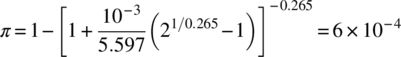By extension, if we further assume a number of individuals are subjected to this exposure, then the expected number of infections may be computed by multiplication. Continuing the example, if 10,000 individuals were exposed to the indicated dose, then it would be anticipated that (6 × 10−4) (10,000) = 6 infections would result. Point estimates for risk can require more than two or three inputs for computation, as indicated in the following example.

The use of point estimates for risk, either the risk per individual or the expected number of adverse outcomes in a population, has the advantage of being simple to compute and being information relatively simple to convey to risk managers. However, it is disadvantageous in that for some (perhaps most) situations, it conveys a false sense of certainty in the computed number. Furthermore, many would presume that if central values are used for each of the inputs to a risk characterization, a central value for the result would occur; similarly that if upper confidence limits for each of the inputs are used, that an upper confidence limit (at a similar confidence level) for the output would be computed. Neither of these presumptions is universally correct. The following illustration addresses the first presumption; a subsequent illustration will address the second presumption.

Consider a risk characterization based on two variables, which we shall call x and y (perhaps one denotes exposure, and the other denotes a potency). The overall risk is estimated as x/y. The input x may take one of five values: <3 × 10−4, 4 × 10−4, 5 × 10−4, 0.001, 0.008>. The input y may take one of three values (independent of x) <0.1, 0.5, 0.7>. The means of x and y are 0.00204 and 0.433, respectively. The medians of x and y are 5 × 10−4 and 0.5, respectively.

If the quantity x/y is evaluated at the median of the two inputs, it is computed to be 0.001, while evaluated at the mean of the two inputs, it is computed to be 0.00471. Now, if the function x/y is evaluated at each of the 15 combinations of x and y, the median and mean of the resulting values can be determined to be 0.002 and 0.00913, respectively. This illustrates that, in general, for a function of random variables (those that can be described as probability distributions), the expected value (mean) is not always equal to the function evaluated at the mean of the inputs, nor is the median equal to the function evaluated at the median of the inputs (nor, in general, is the nth percentile of the result necessarily given by substituting the nth percentile of all of the inputs). The extent of such differences can be computed by the theory of propagation of errors . However, it is presently in general easier to approach the problem by use of the numerical Monte Carlo method. These methods will be discussed subsequently.

Terminology: Types of Uncertainty

Inputs to a risk estimate are uncertain if they are the result of ignorance from “the partial incertitude that arises because of limits on empirical study or mensurational precision” . Empirically, the magnitude of uncertainty, that is, the standard deviation of the distribution used to characterize it, can be diminished by additional effort devoted to measuring that input. These types of uncertainty are often called epistemic (e.g., see Reference ).

In contrast, variability in an input quantity results from an intrinsic heterogeneity in the input. For example, if we were doing a risk estimate (for exposure to infectious agents) among persons going to a beach, we would naturally get a variable exposure (to infectious agents in the water) arising from differing amounts of swimming activity, those who engage in active swimming being more highly exposed than those who do not engage in any water contact, for example. By describing the relative proportions of those engaged in activities leading to different intensities of exposure (again, perhaps, by a probability distribution), we could characterize variability. No amount of additional effort (at measurement) could reduce the magnitude of heterogeneity arising from this differential exposure. Variability is often called aleatory uncertainty (e.g., see Reference ).

Hence, although both uncertain and variable inputs can each be mathematically described by probability distributions, it is important to realize that they are fundamentally addressing different phenomena. Hence, some have advocated dissecting these sources of heterogeneity (in a risk estimate) in the final characterization—which we shall discuss. In fact, a small number of practitioners of risk assessment have argued that probability distributions should not be used at all to describe uncertainty, but rather interval arithmetic (or fuzzy arithmetic) should be used . However, we shall follow a probabilistic framework that appears to have more adherents than the approach using mixed probability distributions and interval arithmetic.

Sources of Uncertainty

There are many sources of uncertainty that may arise in inputs to a risk assessment. These have been categorized into a taxonomy by Finkel . These are arranged schematically in Figure 9.2.

Parameter uncertainty arises from measurement of discrete quantities. In the case of measurement error, physical limitations prevent more precise determinations. For example, in the assessment of exposure to drinking water of necessity, reliance is placed on information on water consumption obtained from questionnaires. There may be imprecision (as well as systematic bias) in the evaluation of this quantity.

Random errors leading to parameter uncertainty typically arise from the use of small data sets. The use of only a small number of MPN assays to characterize microbial density as a component of exposure would be a frequent type of random error. Additionally, the use of small numbers of subjects for dose–response assessment would lead to parameter uncertainty in the dose–response parameters.

Systematic errors arise from inherent flaws in data collection leading to bias in determination of information. In a recreational water situation, if exposure was determined based on microbial densities taken only on samples collected, for example, at 3 pm, there might be a systematic error in estimation with respect to risk to all swimmers if systematic diurnal variability in microbial levels (perhaps from tidal flushing or even inputs from swimmers themselves) existed. Another potential source of systematic error may be the failure to consider the potential for subpopulations that may be more sensitive to, or more adversely impacted by, microbial infections .

Model uncertainty arises from the structural equations or relations used to develop exposure assessment or dose–response assessment. There are several components of model uncertainty that can be further differentiated.

The use of surrogate variables contributes to model uncertainty when there is a potential difference (either random or systematic) between the quantity of interest and the quantity being modeled. A subtle type of use of surrogate variables arises in microbial risk assessment from the different nature of organism enumeration methods employed in dose–response measurement and in environmental sampling. In the development of dose–response relationships, typically pure culture of organisms of high viability is used. In many microbial measurements from environmental media or food, the assessment of viability is impossible, impracticable, or very imprecise. Therefore, the use of “measurable” pathogen levels as surrogates for actual infectious levels presents a source of uncertainty of this type.

Errors from excluded variables arise when there may be factors, not considered in a model, that are in fact significant. For example, if the survival of an organism in food is modeled as a function of pH, water activity, and temperature, this may be used to predict pathogen levels at the point of an exposure [6, 7]. If, in fact, changes in redox potential influence survival and have been excluded from the model, this would represent a potential source of error. This chance of “surprise” is difficult to assess and has not been widely considered from a quantitative point of view.

The presence of abnormal conditions is related to the issue of excluded variables. If we fail to consider the potential for rare but catastrophic failure, then we may fail to account for some aspects contributing to a risk. For example, if we are modeling the risk of microbial illness from drinking water produced from a given water treatment plant, there is a chance (perhaps minor, depending on locale) of treatment failure due to simultaneous failure of a chlorine application system (for disinfection) and the chlorine analyzer (which presumably would alert plant personnel to a performance failure). While we expect that such simultaneous conditions would be extremely rare, they might never be zero, and hence unless we consider such possibilities, we have excluded the contribution of this situation to the resultant total risk.

Uncertainty due to model form may arise in a number of ways. In an exposure assessment, we may have several forms of a relationship characterizing transport. In the assessment of dose–response, we may have a number of plausible dose–response models whose fits are consistent with the data (and again have different behaviors with respect to low-dose extrapolation). Some means to incorporate this possible source of uncertainty may be desirable.

Sources of Variability

Sources of variability arise from identifiable characteristics that result in differential exposure or differential dose–response characteristics. For example, there are systematic differences in food consumption patterns with ethnic group and with region. Hence, we would expect variability due to ethnic group and/or region with respect to microbial risk from particular food items that show such heterogeneity. Similarly, there are differences in drinking water consumption amounts with age and gender .

Variability in dose–response sensitivity may exist, although it is less well documented. Particularly with respect to severity of illnesses, it is known  that there are differential sensitivities to certain organisms in the elderly or the very young. Similarly, the variation in competency of different components of the immunological system as a function of nutrition or other components of health status (such as AIDS or use of immunosuppressant drugs) may alter the intrinsic dose–response or severity responses .

Variability That Is Uncertain

Certain inputs that describe variability among a population exposed may also not be known with certainty, and hence, we can have variability that is uncertain. This may be characterized by two principal aspects or their combination.

We may have variability that is uncertain as to form. For example, the water consumption distribution has ordinarily been described as a lognormal , but perhaps in a particular situation, it may be described adequately by several alternative distributional forms. Especially if a good estimate of upper tail percentiles (e.g., >99%) is required (when those upper percentiles dominate the uncertainty), it may require large number of observations to discriminate among competing distributions .

Variability may also be uncertain with respect to the parameters that characterize the distribution describing variability. For example, consider the food consumption distributions in Table 6.30. The entries (e.g., consumption of eggs) describe distributions obtained from a survey. However, since the survey involved a finite sample, there is statistical uncertainty with respect to the distributional parameters. Whether this uncertainty in variability is substantial enough to impact the results will depend on the size of the underlying survey.

Several authors have described the use of “second-order” random variables to convey information about variability that is uncertain . At this point, the application of such methods is not well reduced to easy utility in terms of available software. However, further discussion of the concept will be presented later.

Approaches to Quantify Parametric Uncertainty

There are several ways to quantify parametric uncertainty for inputs to a risk assessment: likelihood ratio methods, bootstrap, Bayesian methods, and interval methods being the dominant approaches. For determining combined uncertainty resulting from multiple inputs, Monte Carlo methods and Bayesian methods are most common. There is some use of propagation of error methods for simple sensitivity analyses.

Likelihood

In the context of exposure assessment, the likelihood ratio approach was introduced in Chapter 6—see Examples 6.11 and 6.14 and Figure 6.9. The formal criterion is given in Equation (6.64). As shown in Figure 6.9, this results in a series of curves (or surfaces or hypersurfaces, depending upon the number of parameters) that contain within all parameters within a particular confidence region. This technique relies to some degree on the assumption that the underlying model is correct.

Bootstrap

The likelihood method provides a means to estimate the distribution of dose–response parameters. However, when it is desired to estimate uncertainty (or variability) distributions of a parameter that will be used in an overall risk assessment, it is inconvenient because of the relatively difficulty of sampling randomly from that distribution.

An alternative approach to the problem is the use of the bootstrap procedure. In this section, we will present the simple method of bootstrapping observations , which is suitable for simple data such as exposure distributions. A somewhat more complex method suitable for dose–response will be presented subsequently.

The logic of the method is shown in Figure 9.3. The original data is resampled—in other words, if there are 10 original observations, then a new bootstrap sample is constructed by randomly choosing 10 observations from the original data, with replacement (such that one of the original observations might appear in the bootstrap sample more than once). Then, the desired statistic is computed on the bootstrap sample. This process is repeated many times. The distribution of statistics on the ensemble of bootstrap samples is taken as an estimator of what the distribution of the statistic would be in the population from which our sample was drawn.

Other Methods

Bayesian Methods

Bayesian estimation provides an alternative approach to the analysis of data and inference from that data. It can be regarded as an alternative approach to statistical analysis from that presented throughout most of this book. A Bayesian approach can be used to develop an uncertainty distribution for an input to a risk assessment.

The fundamental equation for Bayesian estimation of a set of parameters, Θ, given a set of data, x, is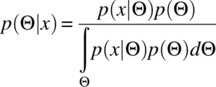In this equation, p(x|Θ) is the probability of obtaining the observed data where the parameter value(s) Θ is true—in other words, this is the ordinary likelihood function defined earlier. p(Θ) is the prior distribution for the parameters, and p(Θ|x) is the posterior distribution of the parameters given the observed data. The posterior distribution can be regarded as the uncertainty distribution. The denominator in Equation (9.2) represents a normalizing factor (integrated over all possible values of Θ) that makes the posterior distribution a proper probability density function. Hence, one can estimate the numerator over all possible values of Θ to obtain the posterior distribution.

The best estimate of Θ is obtained by determining the expectation of the posterior distribution, that is,

(9.3)If Θ has a large number of dimensions, in other words if it contains a large number of individual parameters, then the evaluation of the integral may become complex. For this purpose, various numerical tools such as Markov chain Monte Carlo (MCMC) have become popular. The description of these tools is beyond the scope of the text. For basic information, standard texts (e.g., see Reference ) should be conducted. A variety of software tools, most popularly WinBUGS , are available to facilitate MCMC.

Note that the prior distribution, p(Θ), appears in Equation (9.2). In other words, to estimate the best value of the parameter (set) and the uncertainty distribution, it is necessary to specify the prior. This should be based on an understanding of the preexisting state of knowledge—perhaps from evidence or theory. It may be that minimal or no preexisting knowledge is available, in which case an uninformative prior (which is flat or nearly flat—e.g., a uniform distribution) is used. However, if there is substantial knowledge, a peaked density function may be used. Examples of informative and uninformative priors are shown in Figure 9.4.

If there is only a small amount of data, then the shape of the posterior distribution may closely resemble the prior distribution, and hence the particular form of the prior is quite important. A large amount of data will draw the posterior distribution more substantially away from the prior, and therefore the choice of the prior is less critical. This impact of the specification of the prior on the resulting estimate, which may differ for different analysts, is often a basis of criticism of Bayesian estimation methods.

Applications

Exposure Assessment

Likelihood

Likelihood-based uncertainty distributions for exposure assessment were discussed in Chapter 6. For example, the graph in Figure 6.9 presented the joint uncertainty distribution of the negative binomial parameters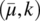describing the coliform data in Table 6.6 and analyzed in Example 6.14.

Generation of this figure requires evaluation of the parameters over a grid and contouring. In principle, it is possible to generate random pairs ofby an acceptance–rejection technique , but it would be computationally tedious:

• Generate a random pair of candidate values, call them (i, j) over a range of possible values, as well as a uniform random number over the interval <0,1>—call this u.
• If (i, j) is within the contour line “u,” then accept (i, j) as a random pair; otherwise reject. We could also use Equation 6.64 to directly determine the acceptance probability of (i, j).

In principle, this method can be used with univariate as well as higher dimensionality distributions, although may be computationally inefficient.

Bootstrap

Typical exposure data, such as those discussed in Chapter 6, consist of repeated measurements of a system—possibly over time or at different locations. If there is presumed to be a systematic variation with time (e.g., seasonality) or with location (“hot spots”), somewhat more complex bootstrap structuring must be used.

Where it can be regarded that systematic temporal or spatial variation can be neglected (or is minor relative to the intrinsic variability between samples), then the set of “N” samples can be subject to be bootstrap by constructing individual bootstrap replicates each consisting of “N” observations drawn from the original data with replacement. From each of the individual bootstrap replicates, the statistic of interest (e.g., sample mean) is computed, and the ensemble of values of that statistic over all of the bootstrap replicates is an estimator of the uncertainty distribution of the statistic.

This is illustrated by reference to the data in Table 6.5, where weekly Cryptosporidium measurements were taken in a water source over the course of a year. Therefore, each bootstrap replicate consists of 52 random draws from the data (note each observation consists in this case of a number of oocysts found and the volume of water that was examined). This computation is especially straightforward in R, which has a bootstrap capability in one of the distributed packages.

The computation is shown in Figure 9.5. This will produce the cdf of the bootstrap estimate of the density of oocysts (defined by the sum of oocyst counts divided by the sum of volumes examined). The result of this computation (for 10,000 bootstrap replicates) is shown in Figure 9.6. The cdf in this figure could be used as an input into an overall risk characterization (or, more directly, the 10,000-individual bootstrapped values of density).

Bayesian

The Bayesian approach to analysis of exposure data is illustrated with reference to the count data (in 1 ml samples) given in Example 6.5. In that example, it was shown that the data were statistically different from what would be predicted from a Poisson distribution. We want to determine the uncertainty of negative binomial parameters that might be used to characterize these data.

The starting point is Equation (9.2). The likelihood function for the unknown parameters (μ,k) given data “x” can be written as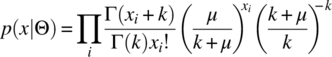Equation (9.4) arises from Equation (6.47) recognizing that the volume in this problem is constant (V = 1 ml for all samples).

To apply a Bayesian approach, we need a prior distribution, p(Θ). If we actually had prior information, we could use an “informative” prior. In this problem, we assume we have been given this data without prior knowledge, so a flat prior over a broad range of parameters is appropriate. So we will assume that the joint prior is independent, that is, the product of the marginal priors:

(9.5)And the marginal priors are given byandWe now evaluate Equation (9.2) by first evaluating the denominator (which is a double integral due to the presence of two parameters). We can then evaluate the posterior distribution, p(Θ|x), at a series of grid points to show the bivariate distribution.

The computations are done in R, which has an available package, cubature, which performs the needed numerical integration. The computation is shown in Figure 9.7.Figure 9.7 R code for determining the posterior distribution for the negative binomial distribution parameters fit to data in Example 6.5.

The resulting contours of the posterior distribution for the negative binomial parameters are shown in Figure 9.8. Note that the contours are “clipped” at the top since the prior distribution, Equation (9.7), for k disallows values greater than 20. This indicates that there is some influence of the prior distribution on the final estimates.2 While this is useful for graphically depicting the spread of parametric uncertainty, for a full risk assessment, it would be useful to draw random samples of the posterior.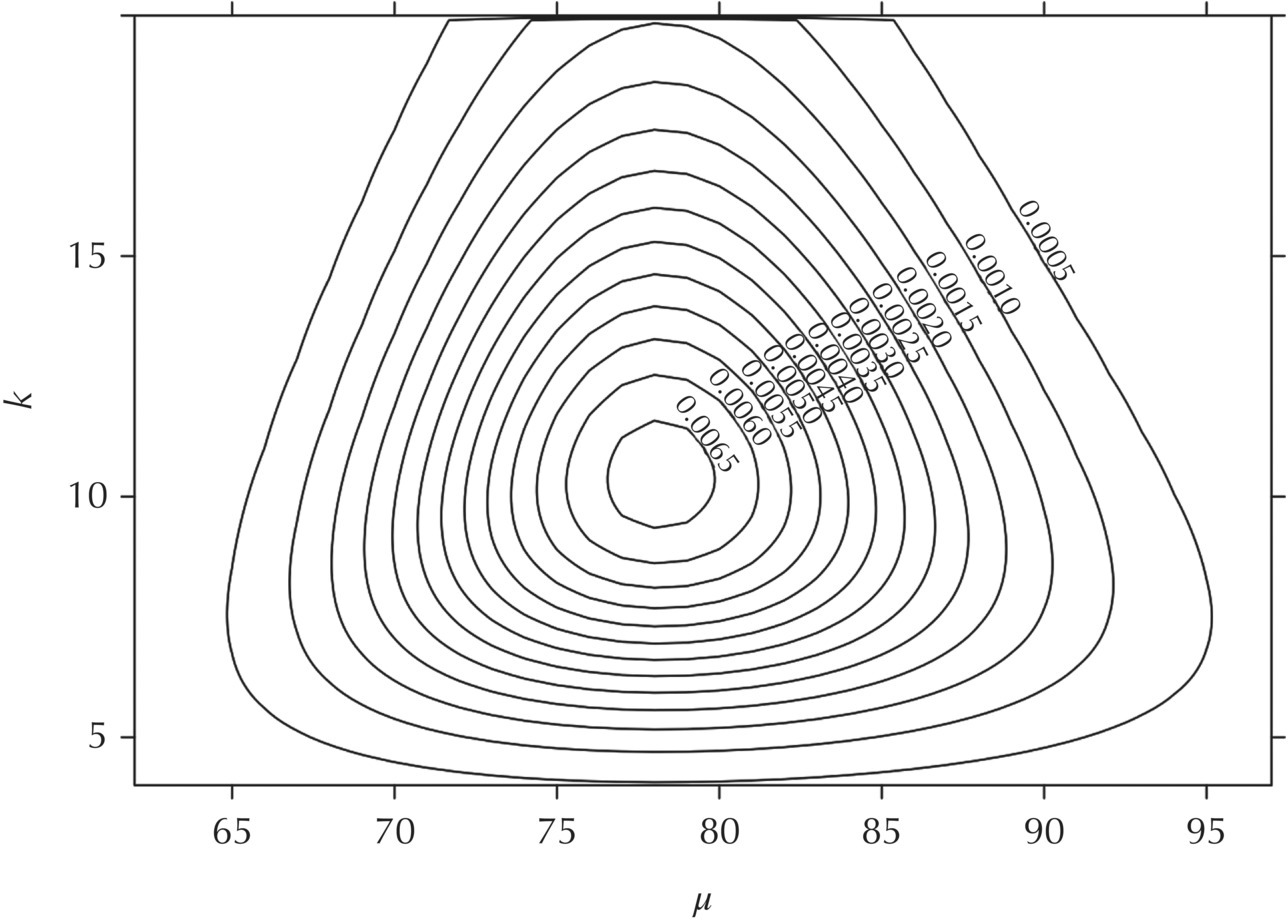Figure 9.8 Contours (numbers indicate values of the density) of the posterior distribution of the negative binomial parameters.

To draw random samples from the posterior, we use a variant of the acceptance–rejection method noted previously—since this is a bivariate distribution, we draw random pairs (μ*, k*) uniformly from the range of possible values (in this case, based on the priors, the ranges indicated in Eqs. (9.6) and (9.7)), as well as a random uniform number, z*. Since the computation of the posterior indicated that no value of the density was in excess of 0.008 (Fig. 9.8), z* is chosen uniformly from the range <0, 0.008>. Then, if the value of the posterior evaluated at (μ*, k*) is greater than z*, the point (μ*, k*) is accepted as one point sampled from the posterior. In Figure 9.9, the code snippet for generating such a sample (which is executed immediately subsequent to the code in Fig. 9.7) is presented.

A set of 2000 samples from the posterior distribution is given in Figure 9.10. To get 2,000 accepted points, over 150,000 random trials needed to be conducted. While this was done sufficiently rapidly (seconds of execution time on a desktop computer), it is inefficient. To achieve greater efficiency, there are particular “tricks” of drawing random numbers from a distribution that more closely envelopes the posterior; however, it may be difficult to ascertain the appropriate distribution. Alternatively, as noted earlier, an MCMC process can be constructed.

Dose–Response Assessment

Likelihood

To assess the precision with which the dose–response curve has been determined, that is, the uncertainty, we would like to determine the confidence limits to the parameters of the dose–response curve and also the upper and lower envelopes (at a certain confidence coefficient) around the dose–response curve.

In the case of a one-parameter model, a direct plot of the excess deviance, Y(Θ) − Y*, versus Θ can be made. This is illustrated in Figure 9.11. From this plot, the 95% confidence interval for the r value of the exponential dose–response model for this data set is determined to be 0.00215–0.00757. Using these values, the upper and lower limits on the dose–response curve are plotted in Figure 8.8.

For dose–response models with more than one parameter, determination of the likelihood-based confidence interval becomes somewhat more complex, since the value of Y(Θ) − Y* must be evaluated over a two- or more dimensional region. The intersection of this surface (or hypersurface) with a level plane (or hyperplane) at the critical χ2 defines the likelihood-based confidence region.

Figure 9.12 presents the confidence contours for the rotavirus (Table 8.4) data set fit to the beta-Poisson model. To obtain these curves, the excess deviance was computed over a two-dimensional grid of α and N50 values. From this, the p values were computed using the cumulative χ2 distribution. Contours were then drawn at specific p values. While this computation can, in principle, be performed using spreadsheets, it is tedious. To produce the graph in Figure 9.12, the MATLAB  program was used to generate the grid of excess deviances. From that, a contouring algorithm can be employed—to produce this graph, the contour routine in the graphical package, IGOR , was used. Similar computations could also be done in R.

If it is desired to estimate the confidence limits on a dose–response curve for a dose–response function with two or more parameters (i.e., similar to the dashed lines in Fig. 8.8), then it is necessary to solve a series of constrained minimization problems of a similar nature to Equation (9.8). For a given dose, d, the upper limit to the dose–response curve is determined from solving the following program (by varying the dose–response parameters, e.g., for beta-Poisson, varying α and N50):And similarly, the lower limit to the dose–response curve is found by minimizing P subject to the indicated constraint. Determination of the full upper and lower limits then requires repeating this computation at various doses and connecting the points thereby obtained.

Figure 9.13 presents the confidence limits to the dose–response curve for rotavirus. Note that the width of the interval tends to increase at lower doses—this is characteristic; in general, the width will increase away from the central point of data. Also note that the confidence limits, as well as the best estimate, tend to become linear with a slope of 1 on a log-log plot (they will become linear on an arithmetic plot as well); this is characteristic of the exponential and beta-Poisson models (as well as all other models that have a linear low-dose property; note though that the log-probit model and the simple threshold models do not have this property).

Bootstrap

The logic of the bootstrap was illustrated in Figure 9.3. The observed dose–response data is the vector of observations (x1, x2, … , xn)—consisting of triplets (mean dose, subjects exposed, positive subjects). This is presumed to be a random sample of some unobserved population of all subjects. From the real data, we compute a statistic—the best-fit value(s) of the dose–response parameters. We want an estimate of what the uncertainty distribution of the dose–response parameters is. By constructing a series of bootstrap replicates (x*), in our case, triplets of (average dose, total subjects, positive subjects), and determining (by maximum likelihood fitting) the distribution of dose–response parameters among the bootstrap replicates, it is inferred that the latter distribution is a good estimator of the uncertainty distribution from the total population in the “real world.” The key question then becomes how to construct bootstrap samples from dose–response data.

This appears not to have been a thoroughly explored subject in the statistical theory of the bootstrap. A review by Hinckley  notes that with respect to bootstrap methodology when the dependent variables are discrete (as in the present case, where they are integers), “More needs to be learned.” The monograph by Efron and Tibshirani  does not include coverage of this type of data (discrete dependent variables). There are at least two different possible methods—termed “bootstrapping observations” and “bootstrapping residuals.”

In the method of “bootstrapping observations,” it is recognized that the “observation” at dose “i” of pi positives out of ni total subjects really represents ni observations out of which pi are positive. Therefore, each bootstrap sample consists of a set of doses for which the positives,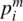(denoting the number of positives at dose level “i” in the mth bootstrap sample), represent a set of ni individuals chosen such that there is a probability pi/ni of being positive. In other words,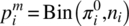(where Bin represents a random sample from a binomial distribution with the given parameters).

As an example, Table 9.1 shows three bootstrap replicates for the Cryptosporidium data set in Table 8.7. The dose and total number of subjects remain constant for all bootstrap replicates, but the original number of positive subjects is replaced by columns A, B, and C (for three replicates). These latter three columns are drawn using Equation (9.9). For each of the bootstrap replicates, the best-fit dose–response model can be determined by the maximum likelihood methods discussed in Chapter 8.

Figure 9.14 presents the results of a run of 1000 bootstrap replications (using the bootstrapping of observations approach) for the rotavirus data (Table 8.4). The bootstrap distribution clearly surrounds the maximum likelihood estimate from the original data and qualitatively covers a similar area as the likelihood-based confidence region. These 1000 replications can then be used as an input to subsequent computations—reflecting the uncertainty distribution to dose–response.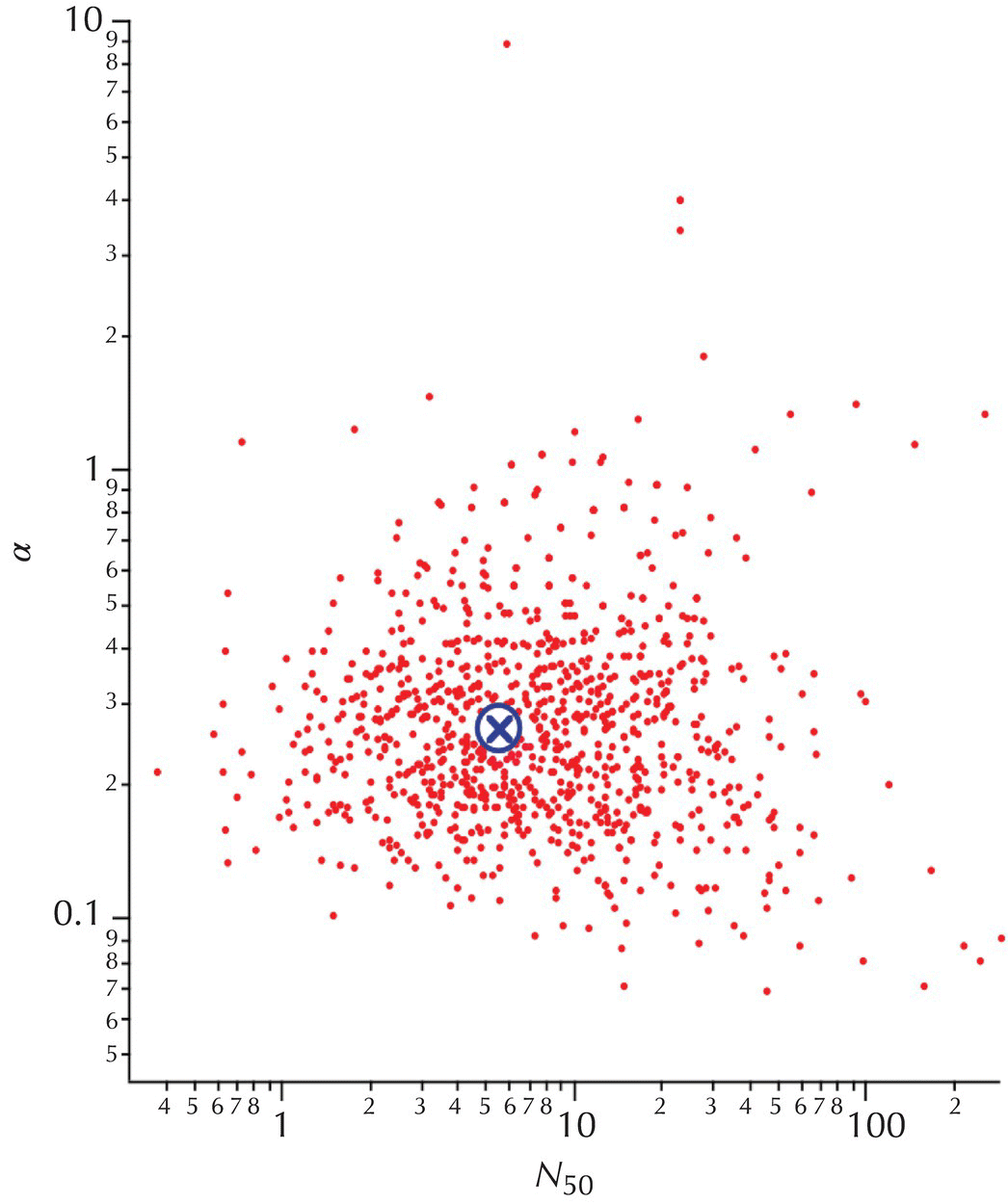Figure 9.14 Bootstrap distribution of rotavirus parameter estimates (beta-Poisson model). Of the 1000 trials, 12 (not plotted) had α > 10. The central marker indicates the maximum likelihood estimate from the underlying data.

The bootstrapped dose–response parameters can also be used to construct alternative confidence regions to the dose–response curve, in comparison to likelihood-based intervals such as in Figure 9.12. At a given dose, each set (say, of the 1000 replicates) is used to compute the estimated response (exponential, beta-Poisson, etc.). Then, to obtain the confidence region (0.95, 0.99, etc.) at a level z, the upper and lower (1 − z)/2 proportions of the responses (at that dose) are found—these define the confidence regions at that concentration.

One of the problems with bootstrapping observations is that by virtue of Equation (9.9), if any of the dose levels have 0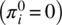or 100%response, then all of the bootstrap replicates will have 0 or 100% response at those dose levels. This might then result in an underestimate of uncertainty in the dose–response parameters.

The method of bootstrapping residuals is one approach to circumvent this particular limitation. A central concept to this method is the definition of a residual from a model fit. Using the definition of a “chi-square” residual, where πi is the estimated proportion of respondents (e.g., infected individuals) at dose i from the dose–response relationship and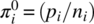is the observed proportion, then the “chi-square” residual is defined by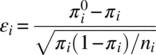For dose–response data, these residuals would be expected to be (asymptotically) normally distributed with zero mean and unit variance .

The first step in execution of the bootstrap procedure is fitting the actual data to the selected dose–response model. This yields a set of values for πi (from the best-fit model) and εi, from Equation (9.10). Next, a pseudosample (bootstrap replicate) is constructed by computingwhere the superscript (m) denotes the mth set of bootstrap replicates, the subscript i refers to the particular dosage within that set, and q is a random index to select one of the ε values defined by Equation (9.10). The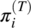values are the best-fit positive proportions to the original data. Since the proportion parameter of the binomial distribution is restricted to values between 0 and 1, the values of the bootstrap pseudoreplicate proportions are adjusted to 0 or 1, if the computations from Equation (9.11) result in negative values or values in excess of 1, respectively. Finally, for each i, given ni and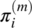, a binomial random number (integer) is drawn to serve as the value for the number of positive responses for dose in bootstrap replication m. In other words, rather than Equation (9.9), Equation (9.12) is used:Now, for each pseudoreplicate, the maximum likelihood fitting process is repeated, such that the best-fit parameter set—generically denoted as Θ(m) (e.g., the pair for the beta-Poisson distribution) is obtained. Then, the parameter sets (for all m, sufficiently large) represent the bootstrap estimate for the uncertainty distribution of the dose–response parameters.

There have not been extensive comparisons of various bootstrap alternatives in dose–response modeling, although preliminary attempts have been made in carcinogen risk assessment .

Regardless of the bootstrapping method used, the “points” of the bootstrap distribution, for example, Figure 9.14, can be used as an input to a full Monte Carlo risk characterization. This will be shown later in this chapter.

Bayesian

Bayesian methods can be used to determine uncertainty distributions for dose–response parameters, analogously to exposure distributions. This requires the specification of a prior distribution for the dose–response parameters, which in the absence of true preexisting knowledge may be uninformative (or flat).

However, a very important use of Bayesian methods appears to be the inferring of the parameters of hyperdistributions from which individual experiments represent a realization. Such analysis is beyond the scope of coverage of this book and would generally require Markov chain methods. However, a few examples illustrate the potential utility of this approach.

Messner et al.  analyzed dose–response data from human studies using multiple strains of Cryptosporidium parvum. It was assumed that each individual strain studied could be characterized as a random strain of possible infectious Cryptosporidium strains and that that set of all possible strains had a hyperdistribution of dose–response parameters. Then, a hierarchical Bayesian analysis was conducted to characterize the parameters of the hyperdistribution. As long as the strains studied could be assumed in fact to be representative of the entire set of strains with respect to infectivity (which is of course unknown), then these hyperdistribution parameters could be used to make inferences about all possible infectious strains.

Mitchell-Blackwood et al.  conducted a similar analysis with respect to a large set of complex animal infectivity studies of Bacillus anthracis spores. In this study, they found evidence that this complex data set might not be best modeled using a single hyperdistribution, but in fact from several hyperdistributions. However, using the single hyperdistribution approach lent support to a judgment of 10,000 spores as being an LD50 (in primates) for B. anthracis.

Combining Parametric Uncertainty from Multiple Sources

Given estimates for the uncertainty or variability from multiple inputs, a risk assessor now desires to combine such estimates into an overall assessment of uncertainty of a function of those individual inputs. There are a number of methods to do this. In this section, these methods are set forth. The use of this process for risk characterization will be presented in Chapter 11.

Propagation Methods

If very little information is known or available with respect to the nature of uncertainty or variability of inputs, then several propagation methods can be used, depending upon how little information is known.

If all that is known of the inputs are the possible upper and lower bounds, then it is only possible to define the upper and lower bounds of any derived function. For example, consider two inputs, X and Y, which are only known to be within an interval:

(9.13)